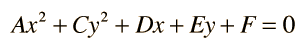# Conic Sections

The curves you get when you slice a cone are called conic sections and have many applications. Click on one for further details.One application is that a moving particle that is subjected to an inverse square law force like gravity or Coulomb's law will follow a path described by one of the conic sections.
 Each of the conic sections can be described in terms of a semimajor axis a and an eccentricity e. Representative values for these parameters are shown along with the types of orbits which are associated with them.Every equation of the formis a conic section except for certain degenerate cases where the equation describes just points or lines. The conditions for the conic sections described above are
• Circle: A and C equal and not zero.
• Ellipse: A and C unequal but have the same sign.
• Parabola: Either A or C (but not both) is zero.
• Hyperbola: A and C have opposite signs
Index

 HyperPhysics*****HyperMath*****Geometry R Nave
Go Back